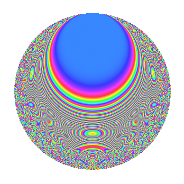# Properties

 Label 1005.2.gLevel 1005 Weight 2 Character orbit g Rep. character $$\chi_{1005}(401,\cdot)$$ Character field $$\Q$$ Dimension 92 Newforms 2 Sturm bound 272 Trace bound 5

# Related objects

## Defining parameters

 Level: $$N$$ = $$1005 = 3 \cdot 5 \cdot 67$$ Weight: $$k$$ = $$2$$ Character orbit: $$[\chi]$$ = 1005.g (of order $$2$$ and degree $$1$$) Character conductor: $$\operatorname{cond}(\chi)$$ = $$201$$ Character field: $$\Q$$ Newforms: $$2$$ Sturm bound: $$272$$ Trace bound: $$5$$

## Dimensions

The following table gives the dimensions of various subspaces of $$M_{2}(1005, [\chi])$$.

Total New Old
Modular forms 140 92 48
Cusp forms 132 92 40
Eisenstein series 8 0 8

## Trace form

 $$92q$$ $$\mathstrut +\mathstrut 96q^{4}$$ $$\mathstrut +\mathstrut 16q^{9}$$ $$\mathstrut +\mathstrut O(q^{10})$$ $$92q$$ $$\mathstrut +\mathstrut 96q^{4}$$ $$\mathstrut +\mathstrut 16q^{9}$$ $$\mathstrut +\mathstrut 72q^{16}$$ $$\mathstrut -\mathstrut 40q^{19}$$ $$\mathstrut -\mathstrut 8q^{21}$$ $$\mathstrut +\mathstrut 32q^{22}$$ $$\mathstrut -\mathstrut 16q^{24}$$ $$\mathstrut +\mathstrut 92q^{25}$$ $$\mathstrut -\mathstrut 40q^{33}$$ $$\mathstrut +\mathstrut 56q^{36}$$ $$\mathstrut -\mathstrut 32q^{37}$$ $$\mathstrut +\mathstrut 24q^{39}$$ $$\mathstrut -\mathstrut 44q^{49}$$ $$\mathstrut -\mathstrut 16q^{54}$$ $$\mathstrut +\mathstrut 16q^{55}$$ $$\mathstrut +\mathstrut 32q^{64}$$ $$\mathstrut -\mathstrut 56q^{67}$$ $$\mathstrut +\mathstrut 16q^{73}$$ $$\mathstrut -\mathstrut 128q^{76}$$ $$\mathstrut +\mathstrut 40q^{81}$$ $$\mathstrut -\mathstrut 24q^{82}$$ $$\mathstrut -\mathstrut 34q^{84}$$ $$\mathstrut +\mathstrut 8q^{88}$$ $$\mathstrut -\mathstrut 18q^{90}$$ $$\mathstrut +\mathstrut 56q^{91}$$ $$\mathstrut +\mathstrut 4q^{93}$$ $$\mathstrut -\mathstrut 98q^{96}$$ $$\mathstrut +\mathstrut O(q^{100})$$

## Decomposition of $$S_{2}^{\mathrm{new}}(1005, [\chi])$$ into irreducible Hecke orbits

Label Dim. $$A$$ Field CM Traces $q$-expansion
$$a_2$$ $$a_3$$ $$a_5$$ $$a_7$$
1005.2.g.a $$46$$ $$8.025$$ None $$0$$ $$0$$ $$-46$$ $$0$$
1005.2.g.b $$46$$ $$8.025$$ None $$0$$ $$0$$ $$46$$ $$0$$

## Decomposition of $$S_{2}^{\mathrm{old}}(1005, [\chi])$$ into lower level spaces

$$S_{2}^{\mathrm{old}}(1005, [\chi]) \cong$$ $$S_{2}^{\mathrm{new}}(201, [\chi])$$$$^{\oplus 2}$$